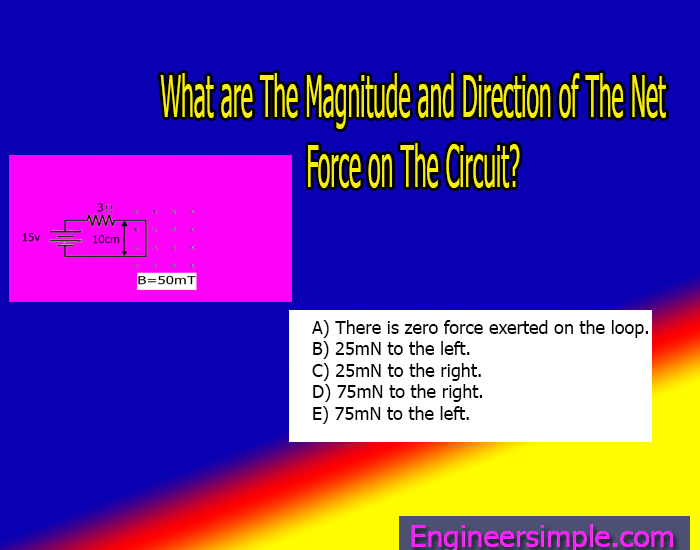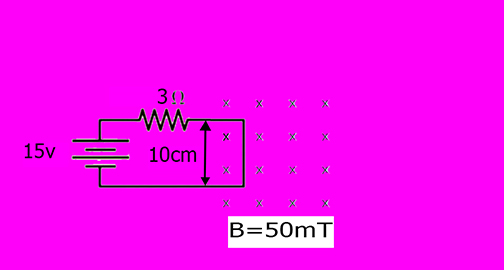What are The Magnitude and Direction of The Net Force on The Circuit? - Engineer Simple

# What are The Magnitude and Direction of The Net Force on The Circuit?

The right edge of the circuit in the Figure extends into a 50mT uniform magnetic field. What are The Magnitude and Direction of The Net Force on The Circuit?A) There is zero force exerted on the loop.
B) 25mN to the left.
C) 25mN to the right.
D) 75mN to the right.
E) 75mN to the left.

Answer: C) 25mN to the right

### Explanation:

Here we get from this circuit,

The magnitude of the Magnetic Field, B= 50mT =50×10-3T

Length, L= 10cm= 0.1m

Potential Difference, V= 15vWe can find the Current by using Ohm’s law,

The angle between the Current element and Magnetic Field, ɵ= 90𑄨

Magnetic Force acting on that segment, F= ILBsinɵ

= 5.0A×0.1m×50×10-3T×sin90𑄨

=25×10-3N

= 25mN

Using Fleming Left Hand Rule,

The Direction of this force is towards the Right.

## Related FAQs

### What is The Net Force of a Circuit?

The net force of a circuit is the force that is exerted on the circuit by the electric and magnetic fields. This force is responsible for the movement of the charges in the circuit.

### How do you calculate the net force of a circuit in a magnetic field?

The net force of a circuit in a magnetic field can be calculated by using the following equation:

F= ILBsinɵ

Where:

F is the net force (in Newtons)

B is the magnetic field strength (in Teslas)

I is the current flowing through the circuit (in Amperes)

L is the length of the circuit (in meters)

This equation can be used to calculate the force exerted by a magnetic field on a current-carrying conductor. The force is perpendicular to both the magnetic field and the current and is given by the product of these two quantities.

No Comment